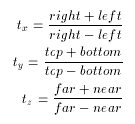# glOrtho

### NAME

glOrtho -- multiply the current matrix by an orthographic matrix

### C SPECIFICATION

```void glOrtho(GLdouble left,
GLdouble right,
GLdouble bottom,
GLdouble top,
GLdouble near,
GLdouble far)
```

### PARAMETERS

left, right
Specify the coordinates for the left and right vertical clipping planes.

bottom, top
Specify the coordinates for the bottom and top horizontal clipping planes.

near, far
Specify the distances to the nearer and farther depth clipping planes. These distances are negative if the plane is to be behind the viewer.

### DESCRIPTION

glOrtho describes a matrix that produces a parallel projection. (left, bottom, -near) and (right, top, -near) specify the points on the near clipping plane that are mapped to the lower left and upper right corners of the window, respectively, assuming that the eye is located at (0, 0, 0). -far specifies the location of the far clipping plane. Both near and far can be either positive or negative. The corresponding matrix iswhereThe current matrix is multiplied by this matrix with the result replacing the current matrix. That is, if M is the current matrix and O is the ortho matrix, then M is replaced with M*O.

Use glPushMatrix and glPopMatrix to save and restore the current matrix stack.

### ERRORS

GL_INVALID_OPERATION is generated if glOrtho is called between a call to glBegin and the corresponding call to glEnd.

### ASSOCIATED GETS

glGet with argument GL_MATRIX_MODE
glGet with argument GL_MODELVIEW_MATRIX
glGet with argument GL_PROJECTION_MATRIX
glGet with argument GL_TEXTURE_MATRIX